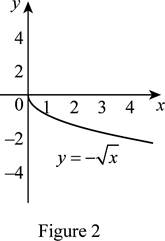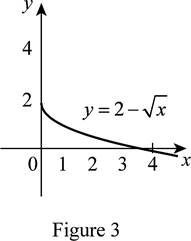# The function y = x by hand without plotting any points and then graph of the function y = 2 − x .### Single Variable Calculus: Concepts...

4th Edition
James Stewart
Publisher: Cengage Learning
ISBN: 9781337687805### Single Variable Calculus: Concepts...

4th Edition
James Stewart
Publisher: Cengage Learning
ISBN: 9781337687805

#### Solutions

Chapter 1.3, Problem 17E
To determine

## To sketch: The function y=x by hand without plotting any points and then graph of the function y=2−x .

Expert Solution

### Explanation of Solution

The standard graph of the function y=x is roughly drawn as shown below in Figure 1.Then, draw the graph of y=x by reflecting the graph y=x about x-axis as it is an odd function. Thus, the graph of y=x is shown below in Figure 2.From Figure 2, it is observed that Figure 1 is reflected about the origin.

To draw the graph of y=2x , shift the graph y=x by 2 units upward. Thus, the graph of y=2x is shown below in Figure 3.Observe that Figure 3is obtainedfrom Figure 1 in such a way that it is reflected about the x-axis and then shifted 2 units upward.

### Have a homework question?

Subscribe to bartleby learn! Ask subject matter experts 30 homework questions each month. Plus, you’ll have access to millions of step-by-step textbook answers!